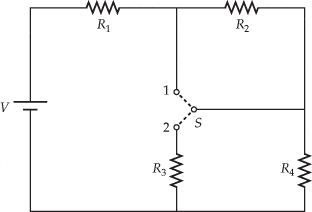# Understanding Short Circuits

I am having trouble understanding short circuits and would appreciate some help with it.

From what I understand, a short circuit occurs when we have a connection with a very low (zero) resistance is made between two points in a circuit. With R=0, the only resistance in our circuit would be the internal resistance of the battery. This causes a large current to go through the circuit Any clarifications, corrections, or additional information is greatly appreciated.

I also had trouble solving a homework problem that uses this concept. The following is the problem:

## Homework Statement

In the circuit below, all four resistors are identical (R1 = R2 = R3 = R4 = R) and the battery has a voltage of 5.17 V.(a) When the switch is placed in position 1, the measured current in the battery is 1.14 mA. What is the value of each resistor?

## Homework Equations

[; I=V/R;]
For resistors in series:
[;R_t_o_t=R_1+R_2;]
For resistors in paraller:
[;R_t_o_t=(1/R_1+1/R_2)^-^1;]

## The Attempt at a Solution

Finding the total resistance of the circuit using Ohm's Law is easy:
[;R_t_o_t=V/I=5.17 V/1.14e^3 A= 4.54e^3 ohm;]

I then use [;R_t_o_t=R_1+R_2+R_3+R_4;] to find an expression for the total resistance in terms of R. Using this second equation with the value found with the first equation allows me to find R.

It turns out that [;R_2;] and [;R_3;] are both equal to zero! This is what I don't understand; WHY are they zero? I believe it has something to do with short circuits but I don't really understand what.

ehild
Homework Helper
I am having trouble understanding short circuits and would appreciate some help with it.

From what I understand, a short circuit occurs when we have a connection with a very low (zero) resistance is made between two points in a circuit. With R=0, the only resistance in our circuit would be the internal resistance of the battery. This causes a large current to go through the circuit Any clarifications, corrections, or additional information is greatly appreciated.

If a resistor is short-circuited, it means connected parallel with a zero resistance: The net resistance becomes zero, the resistor is eliminated from the circuit.

I also had trouble solving a homework problem that uses this concept. The following is the problem:

## Homework Statement

In the circuit below, all four resistors are identical (R1 = R2 = R3 = R4 = R) and the battery has a voltage of 5.17 V.(a) When the switch is placed in position 1, the measured current in the battery is 1.14 mA. What is the value of each resistor?

## Homework Equations

[; I=V/R;]
For resistors in series:
Rtot=R1+R2
For resistors in paraller:
Rtot=(1/R1+1/R2)-1

## The Attempt at a Solution

Finding the total resistance of the circuit using Ohm's Law is easy:

Rtot=V/I=5.17 V/1.14e^-3 A= 4.54e^3 ohm

I then use Rtot=R1+R2+R4 to find an expression for the total resistance in terms of R. Using this second equation with the value found with the first equation allows me to find R.

It turns out that R2 and R3 are both equal to zero! This is what I don't understand; WHY are they zero? I believe it has something to do with short circuits but I don't really understand what.

Redraw the circuit with the switch at position 1. One resistor is short-circuited. Which one? One resistor is not connected into the circuit. Which one?
Why do you think that both R2 and R3 are zero? The resistors are all equal, then the other two would be zero, too. Is it possible?

ehild

Redraw the circuit with the switch at position 1. One resistor is short-circuited. Which one? One resistor is not connected into the circuit. Which one?

Why do you think that both R2 and R3 are zero? The resistors are all equal, then the other two would be zero, too. Is it possible?

ehild

That makes sense. So resistor 3 is not part of the circuit so it is not taken into consideration when calculation the total resistance. Is that correct? But I do not know which one is short circuited, how can I tell?

ehild
Homework Helper
That makes sense. So resistor 3 is not part of the circuit so it is not taken into consideration when calculation the total resistance. Is that correct?

yes, it is correct.

But I do not know which one is short circuited, how can I tell?

The switch is at position 1. You have a single wire connected parallel to resistor R2: it is short-circuited. The wire has zero resistance. The current chooses the zero resistance path, and nothing flows through R2.
(Or you can say that the total resistance of R2 and the zero-resistance wire is zero.)

ehild

#### Attachments

That makes so much sense. So it isn't that R2 and R3 are zero, what happens is that R2 is short circuited and R3 is not connected to the circuit and therefore, they do not contribute any resistance to the total resistance of the circuit! Thank you!!

ehild
Homework Helper
you are welcome.

ehild